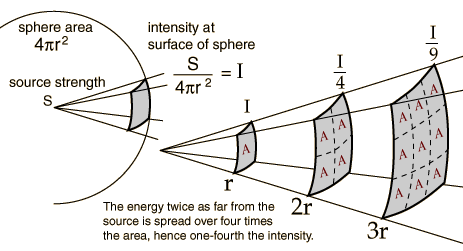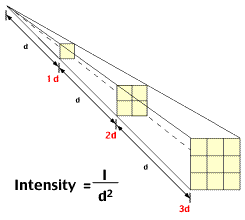EARTHSquare Symbol of EarthAny point source which spreads its influence equally in all directions without a limit to its range will obey the inverse square law. This comes from strictly geometrical considerations. The intensity of the influence at any given radius r is the source strength divided by the area of the sphere. Being strictly geometric in its origin, the inverse square law applies to diverse phenomena. Point sources of gravitational force, electric field, light, sound or radiation obey the inverse square law.

This law accounts for the fact that the intensity of gravitational force, electric field, light, sound or radiation becomes weaker as it spreads out from the source since the same becomes spread over a larger area. The intensity is inversely proportional to the distance from the source.

The Inverse Law states that as a force whether gravitational force, electric field, light, sound or radiation becomes weaker (intensity reduces) as it moves away from its source and spreads.

For example :

An object placed three feet away from a light source will receive only one ninth (1 / 3 2 , the inverse of 3 squared) as much illumination as an object placed one foot from the light.

As a person moves away from source the intensity keeps on decreasing while the surface area increases.Intensity